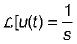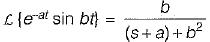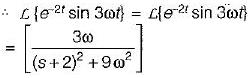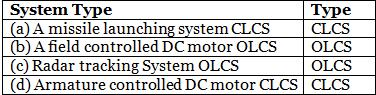Courses

# Introduction to Control Systems - 2

## 10 Questions MCQ Test Control Systems | Introduction to Control Systems - 2

Description
This mock test of Introduction to Control Systems - 2 for Electrical Engineering (EE) helps you for every Electrical Engineering (EE) entrance exam. This contains 10 Multiple Choice Questions for Electrical Engineering (EE) Introduction to Control Systems - 2 (mcq) to study with solutions a complete question bank. The solved questions answers in this Introduction to Control Systems - 2 quiz give you a good mix of easy questions and tough questions. Electrical Engineering (EE) students definitely take this Introduction to Control Systems - 2 exercise for a better result in the exam. You can find other Introduction to Control Systems - 2 extra questions, long questions & short questions for Electrical Engineering (EE) on EduRev as well by searching above.
QUESTION: 1

Solution:
QUESTION: 2

Solution:
QUESTION: 3

### The difference between the output response and the reference signal is known as the _____ signal.

Solution:
QUESTION: 4

The Laplace transform of unity function is

Solution:

The laplace transform of unity function i.e.QUESTION: 5

The phenomena of ‘limit cycles’ and ‘jump resonance’ are observed in

Solution:
QUESTION: 6

The Laplace transform of e-2t sin 3ωt is

Solution:Here, a = 2 and b = 3ωQUESTION: 7

A control system is said to be robust when

Solution:
QUESTION: 8

Which of the following is not correctly matched?
(CLCS = Closed Loop Control System and OLCS = Open Loop Control System)Solution:
QUESTION: 9

Which of the following is not true regarding an open loop control system?

Solution:

The effect of parameter variation and internal noise is more in an open loop system.

QUESTION: 10

As compared to an open loop control system, a closed loop control system

Solution: